# Preprocessing and averaging of multi-channel NIRS data

## Introduction

In this tutorial, you will process a functional near-infrared spectroscopy (fNIRS) data set consisting of multiple channels. We will read in the raw data, have a look at the setup and the data, preprocess the data incorporating specific procedures for multichannel setups, and explore different methods of visualizing the temporal and spatial aspects of the response.

We suggest to first read the Getting started with Artinis page to get the details on the recording system. Furthermore, we suggest you follow the NIRS single channel tutorial to learn how to pre-process a simpler fNIRS data set with only a a single channel.

Note that other NIRS systems will have a different file format than the ones used here and that this tutorial might not directly translate. The global structure however applies to other NIRS systems as well.

## Background

fNIRS is a method to assess changes in oxygenated and deoxygenated hemoglobin concentrations and as such is comparable to fMRI. Disadvantage of fNIRS over fMRI is that it has a (much!) poorer spatial resolution than fMRI, but, the advantage is that it has a better temporal resolution and allows the brain measurements of populations that typically cannot easily be scanned using fMRI. More about the method can be read on the page of the single channel tutorial.

Using multiple channels allows the researcher to investigate whether the found event-related brain activity is local, found in mainly one channel, or more widespread and can be observed in multiple channels. Moreover, the location of the effect can then be determined, though of course one is limited by the spatial resolution of the measurement.

Typically, a detector optode can detect light that originates from multiple source optodes. NIRS systems differ in how this is implemented: for instance, some use slightly different source wavelengths per source optode, others vary the pulse frequency per source optode. Systems also vary in their flexibility: some allow only specific detectors to “look” at specific sources, others allow many more different combinations of sources and optodes. A flexible system can allow you to put for instance a source optode on location x,y, a detector optode on location x+1,y and another detector optode on location x+2,y. Such an arrangement allows the researcher to find proximal, shallow, effects, namely effects observed between the source and detector pair that are positioned next to each other, as well as brain activity somewhat deeper in the brain, namely effects observed between the source and detector pair that are further apart. Combining short channel separations with normal or longer channel separations (channel separation being the distance between source and detector) is a helpful way to cancel out artifacts from among others the skin and motion. Before you can turn to these more sophisticated types of analyses, you need to familiarize yourself with analyzing multiple channels, which is the aim of the current tutorial.

### Dataset information

For the XML file you probably have to right-click and use the save-as option, otherwise it will display the XML content in your browser.

You should now have the following files in your folder:

``````LR-01-2015-06-01-0002.oxy3
LR-02-2015-06-08-0001.oxy3
LR-03-2015-06-15-0001.oxy3
LR-04-2015-06-17-0001.oxy3
LR-05-2015-06-23-0001.oxy3
nirs_48ch_layout.mat
optodetemplates.xml
``````

The participant was engaged in a basic event-related auditory oddball paradigm in an active (target detection) listening situation, while recording changes in oxygenated (HbO2) and deoxygenated hemoglobin (HbR) within left and right temporal cortices. Whenever a tone was presented, a transistor–transistor logic (TTL) pulse was sent to the data acquisition system (the ADC channels of the Oxymon system, see below). This pulse enables us to exactly time and synchronize the stimuli with the fNIRS responses. The stimulus consisted of either a standard 1000 Hz tone or a deviant 1500 Hz tone. Stimuli were presented at intervals of 150 ms, and the deviant was presented as the 3rd to 12th stimulus in a row.

#### fNIRS Measurement

The fNIRS data was recorded using four Oxymon systems from Artinis that were linked to enable a 48 channel recording.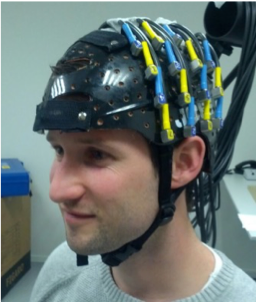Figure 1: Photo of the recording setup.

Half of the optode fibers (n = 16) were placed over left temporal cortex, the other half over the right temporal cortex (Fig. 1 grey text). Of the optodes, half were detectors (or receivers, Rx), and the other half were sources (or transmitters, Tx). The source and detector optodes were positioned such that there were deep and shallow channels of 3 cm and 1.5 cm, respectively (black text indicating both receivers and transmitters).

Sampling was done at 250 Hz. This sampling rate is much higher than needed for NIRS data, so we will downsample the data before starting any fancy analyses.

Trigger events were recorded in the ADC channels 1 (standards) and 2 (deviants). In the Epoch section we will retrieve the onsets and offsets of the trials relative to the triggers.

## Procedure

Analyses can be conducted in many different ways and in different orders, depending on the data and on the experimental design. In the single channel tutorial we introduced you to one order of analysis steps.

The order of steps for this specific tutorial is as follows (see the figure below for an overview):

• optionally downsample the data to reduce memory requirements
• define epochs
• transform optical densities to changes in oxyhemoglobin (oxyHb) and deoxyhemoglobin (deoxyHb) concentration
• separate functional from systemic responses; which can be done using either one of, or a combination of
• filtering; i.e. temporal processing
• subtracting reference channel; i.e. spatial processing (not done here)
• anti-correlating oxyHb/deoxyHb-traces per channel (not done here)
• average the data over trials in each condition
• visualize the results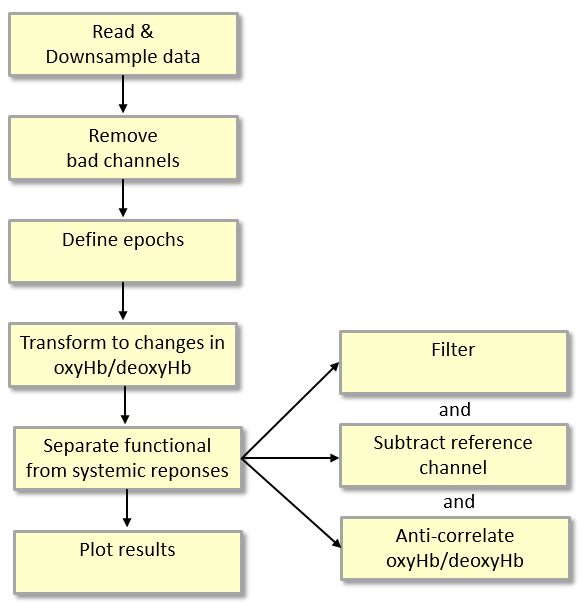Figure 2: Overview of the fNIRS analysis procedure for this tutorial.

We first need to read in the data into the MATLAB workspace, by executing ft_preprocessing:

``````cfg             = [];
cfg.dataset     = 'LR-01-2015-06-01-0002.oxy3';
data_raw        = ft_preprocessing(cfg);
``````

For the purpose of this tutorial, we assume that the data is stored in your current working directory.

Note that the `optodetemplates.xml` file that was included here is a modified version of the default `optodetemplates.xml` that you might have from Artinis. This specific one includes the custom layout of the optodes used in this particular experiment. If MATLAB cannot find the `optodetemplates.xml` on your path, it will pop up a graphical user interface dialogue asking you to locate it. For information on how to choose the optimal template for your experiments, please see this documentation on the Artinis website.

You will see something like this in the command window:

``````processing channel { 'Rx1a-Tx1 [844nm]' ... 'ADC007' 'ADC008' }
reading and preprocessing trial 1 from 1
the call to "ft_preprocessing" took 10 seconds and required the additional allocation of an estimated 732 MB
``````

The structure data_raw contains all data and information about the experiment, all stored in separate fields.

For information about FieldTrip data structures and their fields, see this frequently asked question.

To retrieve the layout from the data file as shown above, you can use:

``````cfg           = [];
cfg.opto      = 'LR-01-2015-06-01-0002.oxy3';
ft_layoutplot(cfg);
``````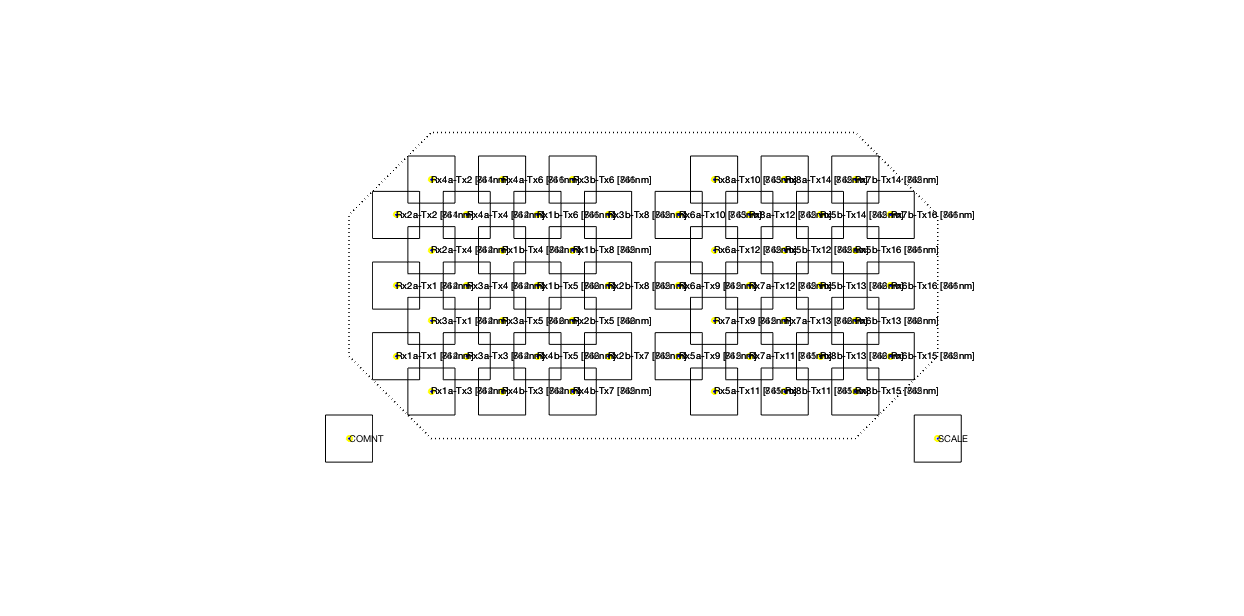Figure 3: Layout of the channels, in between the corresponding optodes.

#### Detecting triggers

The timing of the stimuli is represented in channel ADC001 for the standard tones and in channel ADC002 for the deviant tones. The indices of those channels can be found by looking at the `label` field:

``````find(strcmp(data_raw.label,'ADC001'))
``````

This shows that channel ADC001 is the 97th row in the data matrix, which is stored in data_raw.trial{1}.

Plotting the data from ADC001 and ADC002 will yield the figure below, showing the TTL pulses as analog voltages. Stimulus onset is marked by an abrupt increase in one of the analog channels. Later on, when epoching the data, we will use an automatic routine to find these marked changes.

``````figure; hold on
% increase the scale of ADC002 a little bit to make it more clear in the figure
plot(data_raw.time{1}, data_raw.trial{1}(97,:)*1.0, 'b-')
plot(data_raw.time{1}, data_raw.trial{1}(98,:)*1.1, 'r:')
``````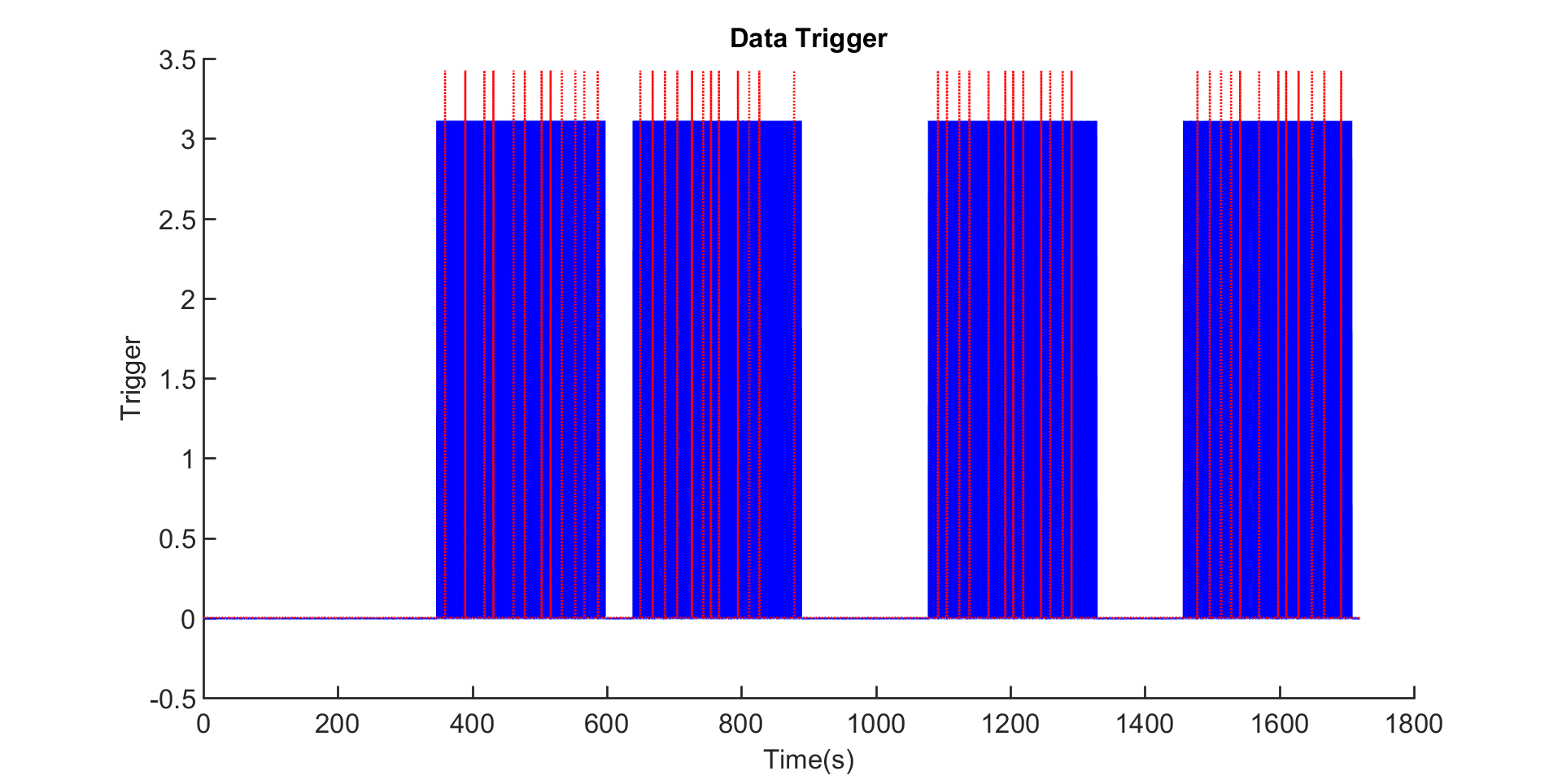Figure 4: Oddball paradigm trigger. All stimuli onsets are indicated by the blue lines. Red dotted lines indicate onsets of the deviants. You can recognize four blocks of events.

Exercise 1: Zoom in on 355 to 365 seconds to better see what is going on. All stimuli onsets are indicated by the blue lines. Red dotted lines indicate onsets of the deviants (the oddballs). Can you now better spot the oddball?

FieldTrip detects the onset in the ADC channels automatically and represents the upward going flank in the ADC channels as events.

``````event = ft_read_event('LR-01-2015-06-01-0002.oxy3')
``````

Exercise 2: Explore the information in the event structure. How many stimuli were played, and how many oddballs? As not all events are stimuli onsets, it might help to select the oddballs with `adc002 = find(strcmp({event.type}, 'ADC002'));`

We will use these events later to define segments of interest and to cut the standard and deviant trials out of the continuous data.

As mentioned, the fNIRS data is stored at 250 Hz. You can check this in

``````data_raw.fsample
``````

Since the hemodynamic response takes about 5 to 10 s to reach its peak (i.e. corresponding to a frequency of 0.2 to 0.1 Hz), a 250 Hz measurement is much faster than needed. To save memory and to make the subsequent processing faster, we will downsample the data to 10 Hz using ft_resampledata.

``````cfg                   = [];
cfg.resamplefs        = 10;
data_down             = ft_resampledata(cfg, data_raw);
``````

If the resampling factor is larger than 10, it is better to resample multiple times. See here.

Downsampling of the slow (compared to EEG and MEG) NIRS signal would normally not be needed, but further down we will segment the data in the reponses to the standard and to the deviant tones. In EEG or MEG the segments of each trial are usually in the order of magnitude of a second or so, and trials do not overlap. In NIRS we need very long segments in the analyusis since the HRF is so slow. The auditory stimuli in this experiment follow each other rapidly, which causes the data segments for the trials to overlap. These overlapping segments are memory inefficient, hence we use downsampling. An alternative would have been to skip the processing of the responses to the standard tones (which we don’t look at anyway) and only process the deviants.

The resampling also includes low-pass filtering of the data. As the new sampling rate is 10 Hz, we will lose data with frequencies larger than 5 Hz. This means we will lose a lot of information from the standards in our experiment, as they are presented near 6.7 Hz, but we keep the deviant information, which is presented near 0.6 Hz. For the current analysis, we are only interested in the deviant data. Just remember: be wary of filtering!

We can now plot the data and see what it looks like. In cfg.preproc we can specify some options for on-the-fly preprocessing. Here, we will demean the data, i.e. subtract the mean value. The options you can specify in cfg.preproc are largely the same as the options for ft_preprocessing with as a difference that in our current command, namely ft_databrowser, the demeaning is only applied for plotting, the data itself remains the same.

``````cfg                = [];
cfg.preproc.demean = 'yes';
cfg.viewmode       = 'vertical';
cfg.continuous     = 'no';
cfg.ylim           = [ -0.003   0.003 ];
cfg.channel        = 'Rx*'; % only show channels starting with Rx
ft_databrowser(cfg, data_down);
``````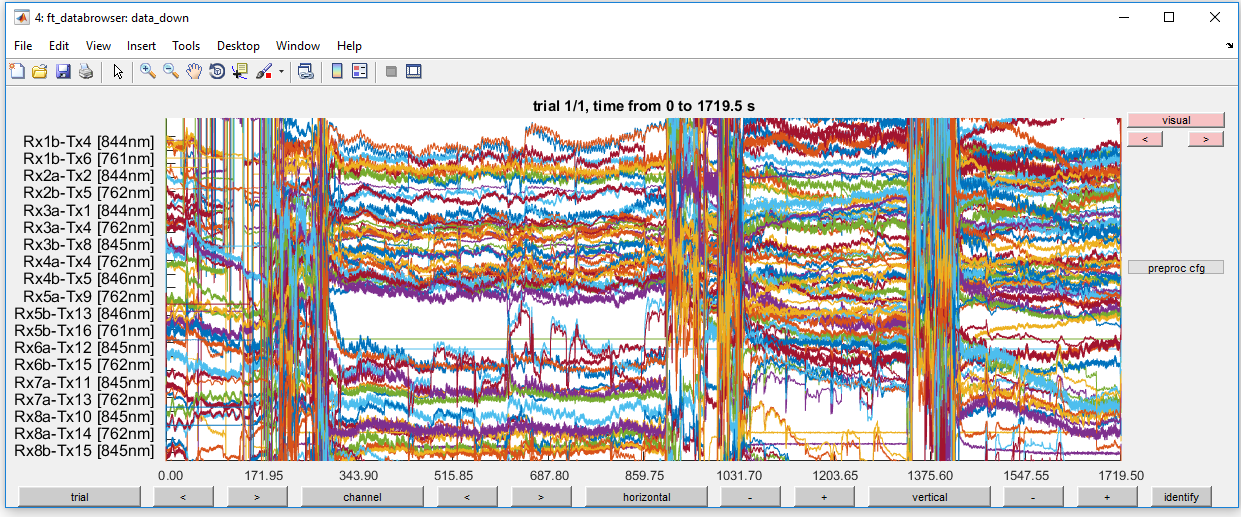Figure 5: Optical density traces for down-sampled data before high-pass filtering.

This is very noisy! Do not give up hope. In the next steps, you will remove most of the noise.

As we are also not interested in very slow changes (and/or a constant offset/ DC) in the hemodynamic response, we can safely throw away low-frequency information by high-pass filtering.

``````cfg                 = [];
cfg.hpfilter        = 'yes';
cfg.hpfreq          = 0.01;
data_flt            = ft_preprocessing(cfg,data_down);
``````

This step has removed some of the variability in the hemodynamic response between channels. Let’s plot the filtered data to see how things have improved.

``````cfg                = [];
cfg.preproc.demean = 'yes';
cfg.viewmode       = 'vertical';
cfg.continuous     = 'no';
cfg.ylim           = [ -0.003   0.003 ];
cfg.channel        = 'Rx*'; % only show channels starting with Rx
ft_databrowser(cfg, data_flt);
``````Figure 6: Optical density traces for down-sampled data after high-pass filtering. Note that the DC (offset) has been largely removed by this step (cf. Fig. 5).

### Epoch

In the single channel tutorial, after initial preprocessing we continued with removing bad data as there were no pieces of the data that were both irrelevant (say, during a break) and very noisy. In this tutorial, we will first segment the data to get the time segments of interest before we move on to cleaning the data further. The motivation here to first segment and then detect artifacts is that the largest artifacts in the data are due to motion artifacts that occur between the experimental blocks. By segmenting the data in trials, these non-relevant sections in the data are ignored and we obtain a cleaner data set already.

In this experiment, the segment of interest is a period of 5 s before and 20s after stimulus onset. We will cut out the segments in the data using the function ft_redefinetrial. Normally we would use ft_definetrial to determine the segments, but due to the resampling the sample indices have changed and hence we will do it by hand.

``````event = ft_read_event('LR-01-2015-06-01-0002.oxy3');

% get the sample number in the original data
% note that we transpose them to get columns

factor = data_raw.fsample / data_down.fsample

% get the sample number after downsampling
smp001 = round((smp001-1)/factor + 1);
smp002 = round((smp002-1)/factor + 1);

pre    =  round( 5*data_down.fsample);
post   =  round(20*data_down.fsample);
offset = -pre; % see ft_definetrial

trl001 = [smp001-pre smp001+post];
trl002 = [smp002-pre smp002+post];

trl001(:,3) = offset;
trl002(:,3) = offset;

trl001(:,4) = 1; % add a column with the condition number
trl002(:,4) = 2; % add a column with the condition number

% concatenate the two conditions and sort them
trl = sortrows([trl001; trl002])

% remove trials that stretch beyond the end of the recording
sel = trl(:,2)<size(data_down.trial{1},2);
trl = trl(sel,:);

cfg     = [];
cfg.trl = trl;
data_epoch = ft_redefinetrial(cfg,data_down);
``````

If you type in data_epoch, you should see this in the command window:

``````data_epoch =

struct with field

hdr: [1x1 struct]
trial: {1x597 cell}
time: {1x597 cell}
fsample: 10
label: {104x1 cell}
opto: [1x1 struct]
trialinfo: [597x1 double]
sampleinfo: [597x2 double]
cfg: [1x1 struct]
``````

Notably, both trial and time fields will now have 1x597 cell-array (compare this to data_down). This corresponds to the 597 stimuli that were presented. In data_epoch.trialinfo the information about the type of stimulus is stored (event 1 or event 2). Thus, we can find which of those cells belongs to the first deviant:

``````idx = find(data_epoch.trialinfo==2, 1, 'first')
``````

which should give you:

``````idx =

8
``````

Let’s take a look at what happens around the first deviant, by plotting the average optical density:

``````cfg          = [];
cfg.channel  = 'Rx*';
cfg.trials   = 8;
cfg.baseline = 'yes';
ft_singleplotER(cfg, data_epoch)
``````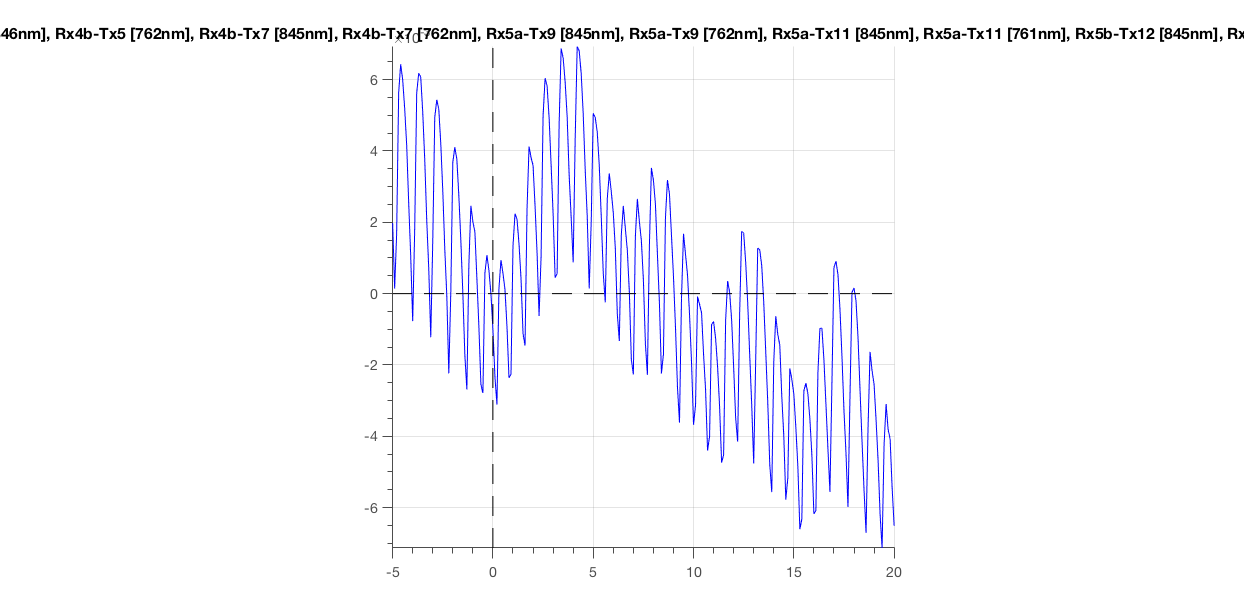Figure 7: Epoched optical density data around the first deviant stimulus.

The most obvious thing you should see, is the heartbeat. This is great! It means that your subject is alive and has some blood flowing through his/her brain (or skin). Importantly, this is an indicative sign of a good measurement. If you would not see this, you could throw this data in the bin (see next paragraph).

Exercise 3: Inspect the signal carefully! When does it increase/decrease, when does it peak? Could this be a functional response? We have to do a few more additional analysis steps, before we know for sure.

First, we will remove the optode channels that make poor contact with the skin of the scalp yielding bad signal because of that. From the optical density traces we can estimate whether there is a good coupling between optode and scalp, because the two signals from each optode (corresponding to the two wavelengths) should have a heartbeat that is positively correlated. If the correlation is small or negative, we exclude that optode from further processing. This is implemented in ft_nirs_scalpcouplingindex. For more details see Polloniniet al. (2014), Auditory cortex activation to natural speech and simulated cochlear implant speech measured with functional near-infrared spectroscopy.

``````cfg      = [];
data_sci = ft_nirs_scalpcouplingindex(cfg, data_epoch);
``````

You can see that you throw away some channels in data_sci.label, where we now only have 86 labels instead of 104:

``````data_sci =

struct with field

hdr: [1x1 struct]
trial: {1x597 cell}
time: {1x597 cell}
fsample: 10
label: {86x1 cell}
opto: [1x1 struct]
trialinfo: [597x1 double]
sampleinfo: [597x2 double]
cfg: [1x1 struct]
``````

### Remove artefacts

We already removed major motion artifacts by epoching, thus removing the periods in between blocks, and by throwing away poorly coupled optodes. Therefore, this step can be ignored for this dataset.

Exercise 4: We just wrote “Therefore, this step can be ignored.” Check this yourself, are there indeed no artifacts? Hint: you can use `cfg.artfctdef.zvalue.interactive = 'yes';` and `[cfg, artifact] = ft_artifact_zvalue(cfg, data_epoch);` like in Exercise 2 of the single channel tutorial.

### Transform optical densities to oxy- and deoxy-hemoglobin concentration changes

Like in the single channel tutorial, we will now convert the optical densities into oxygenated and deoxygenated hemoglobin concentrations by using ft_nirs_transform_ODs.

``````cfg                 = [];
cfg.target          = {'O2Hb', 'HHb'};
cfg.channel         = 'nirs'; % e.g., one channel incl. wildcards, you can also use ?all? to select all NIRS channels
data_conc           = ft_nirs_transform_ODs(cfg, data_sci);
``````

Check the data again using ft_singleplotER. You should see a clear heartbeat in the signal.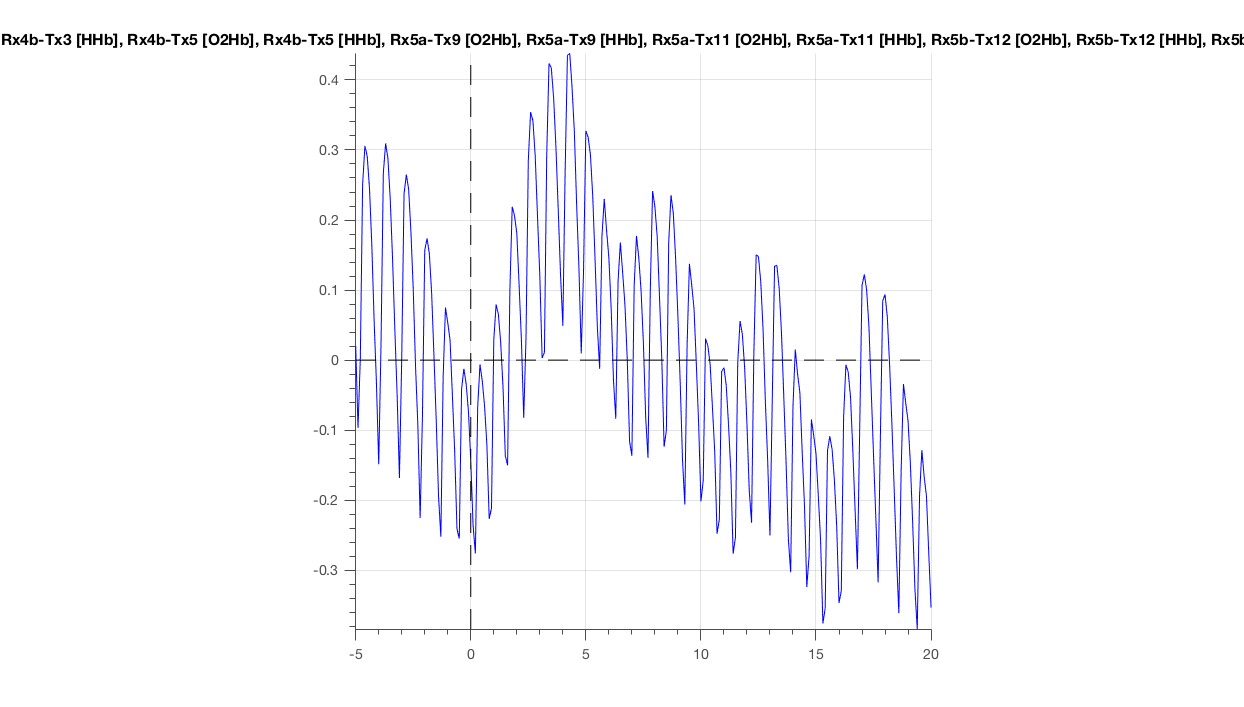Figure 8: Hemoglobin concentration as a function of time, averaged over all channels for the epoch around the first deviant.

### Separate functional from systemic responses

#### Low-pass filtering

The heartbeat is not a signal that we are currently interested in, although you might be if you are interested in effort or exertion. To suppress the heartbeat, we will low-pass filter our data below the frequency of the heart beat (around 1 Hz).

``````cfg                   = [];
cfg.lpfilter          = 'yes';
cfg.lpfreq            = 0.8;
data_lpf              = ft_preprocessing(cfg, data_conc);
``````

The changes in average concentration now reveals a perfect example of the hemodynamic response. No heartbeat, the signal starts to rise at stimulus onset, peaks at around 4 s, and then drops again. Note that the absolute values also make sense (0.37 for the peak).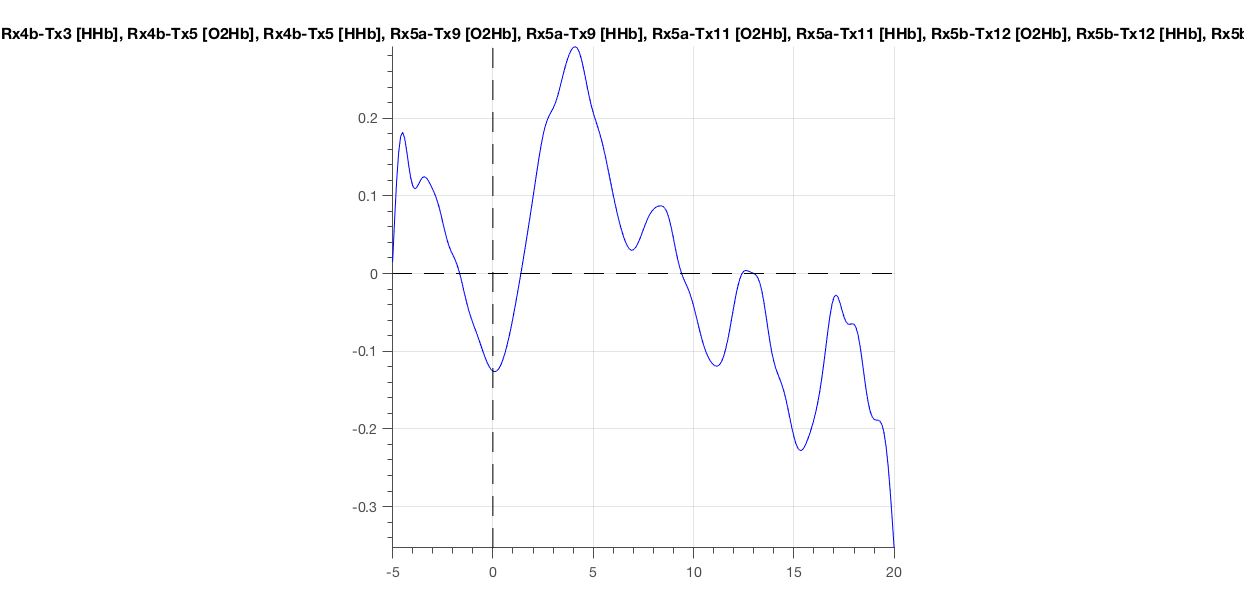Figure 9: Low-pass filtered hemoglobin concentrations (cf. two previous figs.).

### Plot results

Now that we obtained the functional responses, the next step is to average over trials and to visualize the results. First, we will run ft_timelockanalysis to compute the average. The default behavior of the ft_timelockanalysis is to average across all trials. We want to make a separate average for the deviant and for the standard trials, hence we need inform the code which trials belong to the standard stimuli and which belong to the deviants. Information about the conditions is stored in the trialinfo, where 1 represents the standards, and 2 represents the deviants.

``````cfg               = [];
cfg.trials        = find(data_lpf.trialinfo(:,1) == 1);
timelockSTD       = ft_timelockanalysis(cfg, data_lpf);
``````

Then, we will apply a baseline correction using ft_timelockbaseline. The five seconds preceding the stimulus will be used as time window for the baseline.

``````cfg                 = [];
cfg.baseline        = [-5 0];
timelockSTD         = ft_timelockbaseline(cfg, timelockSTD);
``````

In the previous steps, you averaged over all standard trials and baseline corrected the result. The same can be done for the deviants.

``````cfg           = [];
cfg.trials    = find(data_lpf.trialinfo(:,1) == 2);
timelockDEV   = ft_timelockanalysis(cfg, data_lpf);

cfg           = [];
cfg.baseline  = [-5 0];
timelockDEV   = ft_timelockbaseline(cfg, timelockDEV);
``````

To visualize the data in spatial terms (i.e. to answer the question “where on the head do we find functional brain activity in response to my different conditions?”), FieldTrip requires information about the spatial layout about the location of the channel on the head. For this tutorial a layout file is provided, which is called `nirs_48ch_layout.mat`. The layout tutorial explains how to create your own channel layout for plotting, and we have a NIRS layout example that shows it in detail.

The channel layout can be read the `nirs_48ch_layout.mat` file using the standard MATLAB function `load`. The file contains a structure called `lay`. The channel layout has been designed to show the O2Hb and HHb channels on top of each other.

``````load('nirs_48ch_layout.mat')
figure; ft_plot_layout(lay) % note that O2Hb and HHb channels fall on top of each other
``````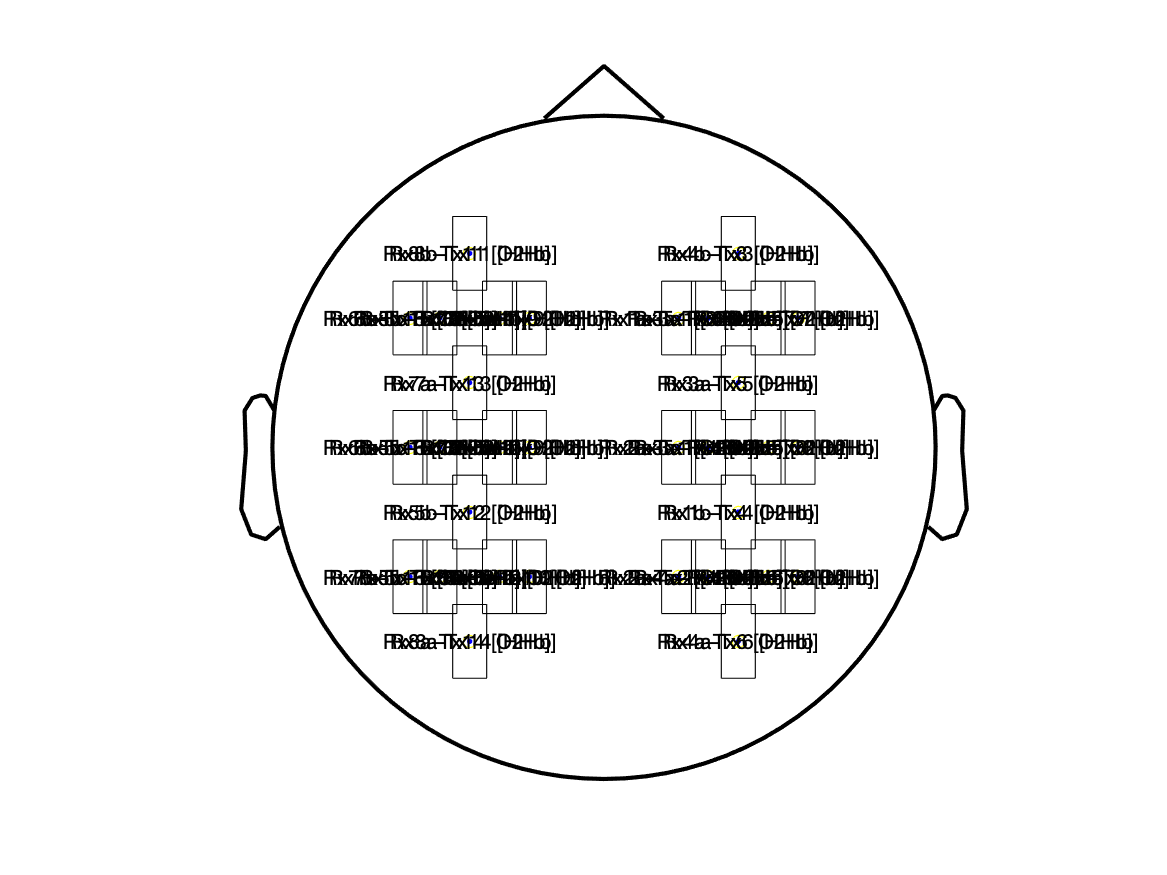Figure 10: Channel layout for multiplot and topoplot.

There are a number of FieldTrip options available for visualizing the results, such as ft_singleplotER (ER stands for event-related), which allows you to plot a single channel, and ft_multiplotER, which allows you to plot multiple channels on a schematic representation of the head. The ft_multiplotER can also be used in interactive mode to select pieces of the data of interest (for instance specific channels and a specific time window).

Important to remember is that for ft_multiplotER to run, you need to point FieldTrip to the layout structure using `cfg.layout = lay`.

``````cfg                   = [];
cfg.showlabels        = 'yes';
cfg.layout            = lay;      % you could also specify the name of the mat file
cfg.interactive       = 'yes';
cfg.linecolor         = 'rb';
cfg.colorgroups(contains(timelockDEV.label, 'O2Hb')) = 1; % these will be red
cfg.colorgroups(contains(timelockDEV.label, 'HHb'))  = 2; % these will be blue
ft_multiplotER(cfg, timelockDEV);
``````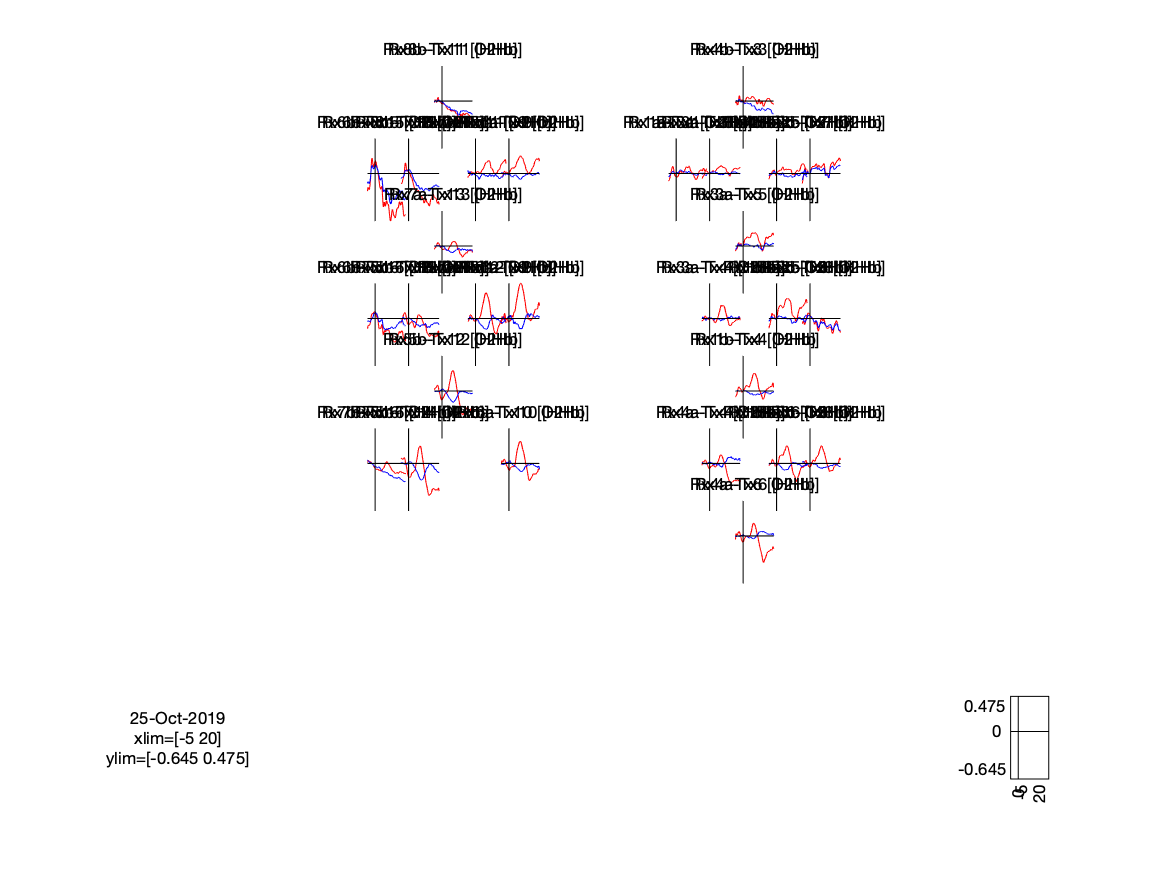Figure 11: A so-called multiplot of the data: the average time course displayed per channel.

You can also generate a spatial representation of the signal at a certain time point, or averaged over a time window. To plot the response that was found during a specific time window, you will need to specify this by setting limitations to the time dimension. The time window can be set by using `cfg.xlim = [5 7];`. The scale for the strength of the response can be set from -0.2 to 0.2, but this depends on your data: many fNIRS researchers use block designs, and depending on the block duration, the response may gain a larger amplitude. In the current data, the scale can be derived from the previous figure, which was generated an automatic scaling of the response amplitude.

Per default FieldTrip uses the minimum and the maximum in the selected part of the data for the `zlim` parameter. Setting the scale manually has the advantage that you can set zero as the middle point in the scale, which can be helpful for the interpretation of the color-coded graph.

``````cfg          = [];
cfg.layout   = lay;      % you could also specify the name of the mat file
cfg.marker   = 'labels';
cfg.xlim     = [5 7];
cfg.zlim     = [-0.2 0.2];

cfg.channel  = '* [O2Hb]';
figure; subplot(1,2,1);
ft_topoplotER(cfg, timelockDEV);
title('[O2Hb]');

cfg.channel  = '* [HHb]';
subplot(1,2,2);
ft_topoplotER(cfg, timelockDEV);
title('[HHb]');
``````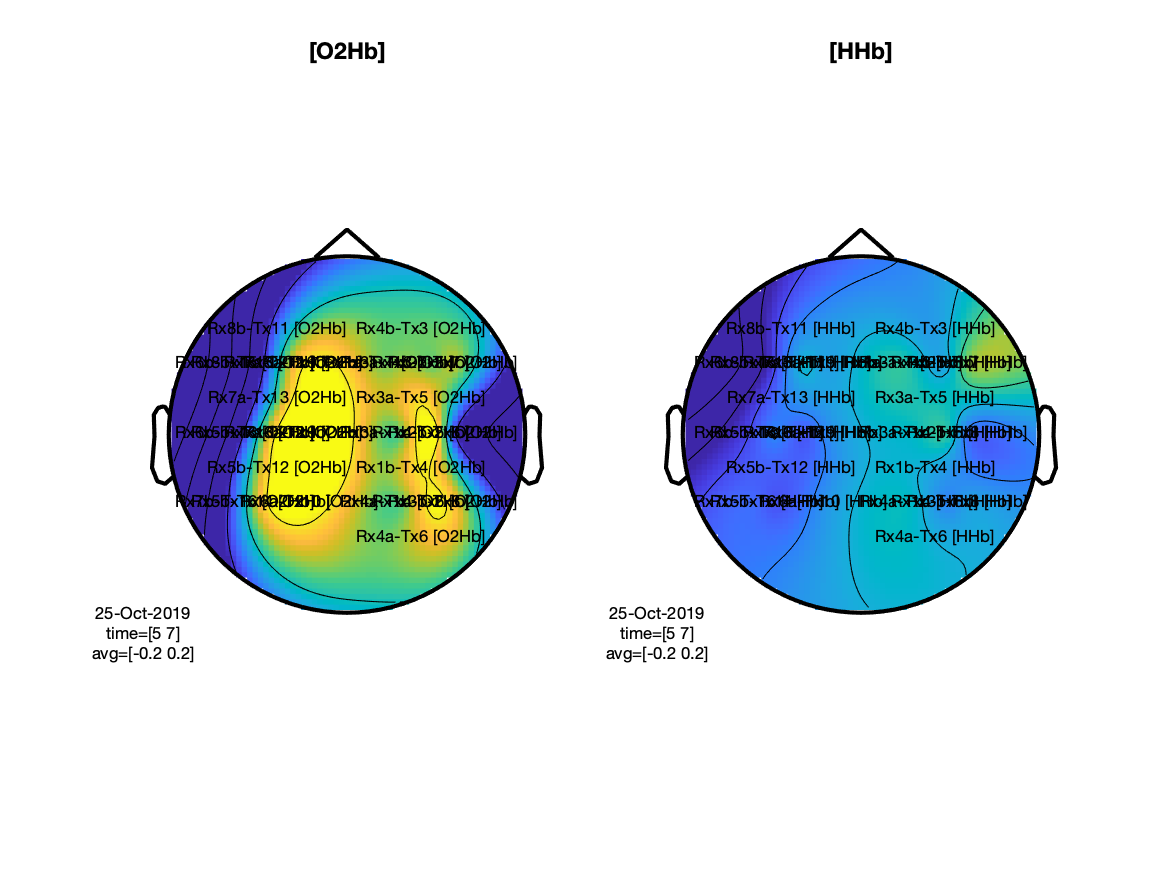Figure 12: Topographical representation of the oxi- and deoxy-hemoglobin signal changes following the deviant tone.

## Summary and conclusion

In this tutorial we have processed a functional near-infrared spectroscopy data set consisting of multiple channels.